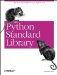# The math Module

The math module implements a number of mathematical operations for floating-point numbers. The functions are generally thin wrappers around the platform C library functions of the same name, so results may vary slightly across platforms in normal cases, or vary a lot in exceptional cases. Example 1-60 demonstrates the use of the math module.

Example 1-60. Using the math Module

```File: math-example-1.py

import math

print "e", "=>", math.e
print "pi", "=>", math.pi
print "hypot", "=>", math.hypot(3.0, 4.0)

# and many others...

e => 2.71828182846
pi => 3.14159265359
hypot => 5.0```

See the Python Library Reference for a full list of functions.Python Standard Library (Nutshell Handbooks) with
ISBN: 0596000960
EAN: 2147483647
Year: 2000
Pages: 252
Authors: Fredrik Lundh